Discrete Mathematics and Combinatorics Commons™

1,040 Full-Text Articles 1,172 Authors 263,379 Downloads107 Institutions

All Articles in Discrete Mathematics and Combinatorics

1,040 full-text articles. Page 1 of 40.

Generating B-Nomial Numbers, 2022Shippensburg University of Pennsylvania

Generating B-Nomial Numbers, Ji Young Choi

Communications on Number Theory and Combinatorial Theory

This paper presents three new ways to generate each type of b-nomial numbers: We develop ordinary generating functions, we find a whole new set of recurrence relations, and we identify each b-nomial number as a single binomial coefficient or as an alternating sum of products of two binomial coefficients.

Hurwitz Actions On Reflection Factorizations In Complex Reflection Group G6, 2021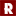George Washington University

Hurwitz Actions On Reflection Factorizations In Complex Reflection Group G6, Gaurav Gawankar, Dounia Lazreq, Mehr Rai, Seth Sabar

We show that in the complex reflection group G6, reflection factorizations of a Coxeter element that have the same length and multiset of conjugacy classes are in the same Hurwitz orbit. This confirms one case of a conjecture of Lewis and Reiner.

A Complete Characterisation Of Vertex-Multiplications Of Trees With Diameter 5, 2021National Institute of Education, Nanyang Technological University of Singapore

A Complete Characterisation Of Vertex-Multiplications Of Trees With Diameter 5, Willie Han Wah Wong, Eng Guan Tay

Theory and Applications of Graphs

For a connected graph $G$, let $\mathscr{D}(G)$ be the family of strong orientations of $G$; and for any $D\in\mathscr{D}(G)$, we denote by $d(D)$ the diameter of $D$. The $\textit{orientation number}$ of $G$ is defined as $\bar{d}(G)=\min\{d(D)\mid D\in \mathscr{D}(G)\}$. In 2000, Koh and Tay introduced a new family of graphs, $G$ vertex-multiplications, and extended the results on the orientation number of complete $n$-partite graphs. Suppose $G$ has the vertex set $V(G)=\{v_1,v_2,\ldots, v_n\}$. For any sequence of $n$ positive integers ...

Rippled Almost Periodic Behavior In An Epilepsy Model, 2021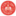VCU

Rippled Almost Periodic Behavior In An Epilepsy Model, David Chan, Candace Kent

Annual Symposium on Biomathematics and Ecology Education and Research

No abstract provided.

2021University of Dayton

Identification Of Control Targets In Boolean Networks Via Computational Algebra, Alan Veliz-Cuba

Annual Symposium on Biomathematics and Ecology Education and Research

No abstract provided.

Building Model Prototypes From Time-Course Data, 2021University of Kentucky

Building Model Prototypes From Time-Course Data, David Murrugarra, Alan Veliz-Cuba

Annual Symposium on Biomathematics and Ecology Education and Research

No abstract provided.

Distribution Of The P-Torsion Of Jacobian Groups Of Regular Matroids, 2021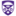The University of Western Ontairo

Distribution Of The P-Torsion Of Jacobian Groups Of Regular Matroids, Sergio R. Zapata Ceballos

Electronic Thesis and Dissertation Repository

Given a regular matroid $M$ and a map $\lambda\colon E(M)\to \N$, we construct a regular matroid $M_\lambda$. Then we study the distribution of the $p$-torsion of the Jacobian groups of the family $\{M_\lambda\}_{\lambda\in\N^{E(M)}}$. We approach the problem by parameterizing the Jacobian groups of this family with non-trivial $p$-torsion by the $\F_p$-rational points of the configuration hypersurface associated to $M$. In this way, we reduce the problem to counting points over finite fields. As a result, we obtain a closed formula for the proportion of these groups ...

Introduction To Discrete Mathematics: An Oer For Ma-471, 2021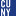CUNY Queensborough Community College

Introduction To Discrete Mathematics: An Oer For Ma-471, Mathieu Sassolas

Open Educational Resources

The first objective of this book is to define and discuss the meaning of truth in mathematics. We explore logics, both propositional and first-order , and the construction of proofs, both formally and human-targeted. Using the proof tools, this book then explores some very fundamental definitions of mathematics through set theory. This theory is then put in practice in several applications. The particular (but quite widespread) case of equivalence and order relations is studied with detail. Then we introduces sequences and proofs by induction, followed by number theory. Finally, a small introduction to combinatorics is given.

Upper Bounds For Inverse Domination In Graphs, 2021Clayton State University

Upper Bounds For Inverse Domination In Graphs, Elliot Krop, Jessica Mcdonald, Gregory J. Puleo

Theory and Applications of Graphs

In any graph $G$, the domination number $\gamma(G)$ is at most the independence number $\alpha(G)$. The \emph{Inverse Domination Conjecture} says that, in any isolate-free $G$, there exists pair of vertex-disjoint dominating sets $D, D'$ with $|D|=\gamma(G)$ and $|D'| \leq \alpha(G)$. Here we prove that this statement is true if the upper bound $\alpha(G)$ is replaced by $\frac{3}{2}\alpha(G) - 1$ (and $G$ is not a clique). We also prove that the conjecture holds whenever $\gamma(G)\leq 5$ or $|V(G)|\leq 16$.

Skolem Number Of Cycles And Grid Graphs, 2021Southern Connecticut State University

Skolem Number Of Cycles And Grid Graphs, Braxton Carrigan, John Asplund

Theory and Applications of Graphs

A Skolem sequence can be thought of as a labelled path where two vertices with the same label are that distance apart. This concept has naturally been generalized to labellings of other graphs, but always using at most two of any integer label. Given that more than two vertices can be mutually distance d apart, we define a new generalization of a Skolem sequences on graphs that we call proper Skolem labellings. This brings rise to the question; what is the smallest set of consecutive positive integers we can use to proper Skolem label a graph?'' This will be known ...

Domino Circles, 2021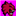Bard College

Domino Circles, Lauren L. Rose, A. Gwinn Royal, Amanda Serenevy, Anna Varvak

Journal of Math Circles

Creating a circle with domino pieces has a connection with complete graphs in Graph Theory. We present a hands-on activity for all ages, using dominoes to explore problem solving, pattern recognition, parity, graph theory, and combinatorics. The activities are suitable for elementary school students, the graph theory interpretations are suitable for middle and high school students, and the underlying mathematical structures will be of interest to college students and beyond.

An Upper Bound On The Spectral P-Norms Of Tensors And Matrix Permanent, 2021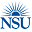Nova Southeastern University

An Upper Bound On The Spectral P-Norms Of Tensors And Matrix Permanent, Killian J. Hitsman, Vehbi E. Paksoy

No abstract provided.

Categorical Aspects Of Graphs, 2021Western University

Categorical Aspects Of Graphs, Jacob D. Ender

In this article, we introduce a categorical characterization of directed and undirected graphs, and explore subcategories of reflexive and simple graphs. We show that there are a number of adjunctions between such subcategories, exploring varying combinations of graph types.

Spectral Graph Theory And Research, 2021Western University

Spectral Graph Theory And Research, Nathan H. Kershaw, Lewis Glabush

Our topic of study was Spectral Graph Theory. We studied the algebraic methods used to study the properties of graphs (networks) and became familiar with the applications of network analysis. We spent a significant amount of time studying the way virus’s spread on networks, with particular applications to Covid-19. We also investigated the relationship between graph spectra and structural properties.

On \Delta^(K)-Colouring Of Powers Of Paths And Cycles, 2021Christ University, Bangalore

On \Delta^(K)-Colouring Of Powers Of Paths And Cycles, Merlin Thomas Ellumkalayil Ms, Sudev Naduvath

Theory and Applications of Graphs

In a proper vertex colouring of a graph, the vertices are coloured in such a way that no two adjacent vertices receive the same colour, whereas in an improper vertex colouring, adjacent vertices are permitted to receive same colours subjected to some conditions. An edge of an improperly coloured graph is said to be a bad edge if its end vertices have the same colour. A near proper colouring is a colouring which minimises the number of bad edges by restricting the number of colour classes that can have adjacency among their own elements. The $\delta^{(k)}$- colouring is a ...

On Graphs With Proper Connection Number 2, 2021University of Alaska Fairbanks

On Graphs With Proper Connection Number 2, Jill Faudree, Leah Berman, Glenn Chappell, Chris Hartman, John Gimbel, Gordon Williams

Theory and Applications of Graphs

An edge-colored graph is properly connected if for every pair of vertices u and v there exists a properly colored uv-path (i.e. a uv-path in which no two consecutive edges have the same color). The proper connection number of a connected graph G, denoted pc(G), is the smallest number of colors needed to color the edges of G such that the resulting colored graph is properly connected. An edge-colored graph is flexibly connected if for every pair of vertices u and v there exist two properly colored paths between them, say P and Q, such that the first ...

2021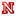University of Nebraska-Lincoln

A Combinatorial Formula For Kazhdan-Lusztig Polynomials Of Sparse Paving Matroids, George Nasr

Dissertations, Theses, and Student Research Papers in Mathematics

We present a combinatorial formula using skew Young tableaux for the coefficients of Kazhdan-Lusztig polynomials for sparse paving matroids. These matroids are known to be logarithmically almost all matroids, but are conjectured to be almost all matroids. We also show the positivity of these coefficients using our formula. In special cases, such as for uniform matroids, our formula has a nice combinatorial interpretation.

Bootstrap Percolation On Random Geometric Graphs, 2021University of Nebraska - Lincoln

Bootstrap Percolation On Random Geometric Graphs, Alyssa Whittemore

Dissertations, Theses, and Student Research Papers in Mathematics

Bootstrap Percolation is a discrete-time process that models the spread of information or disease across the vertex set of a graph. We consider the following version of this process:

Initially, each vertex of the graph is set active with probability p or inactive otherwise. Then, at each time step, every inactive vertex with at least k active neighbors becomes active. Active vertices will always remain active. The process ends when it reaches a stationary state. If all the vertices eventually become active, then we say we achieve percolation.

This process has been widely studied on many families of graphs, deterministic ...

Partially Oriented 6-Star Decomposition Of Some Complete Mixed Graphs, 2021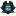East Tennessee State University

Partially Oriented 6-Star Decomposition Of Some Complete Mixed Graphs, Kazeem A. Kosebinu

Electronic Theses and Dissertations

Let $M_v$ denotes a complete mixed graph on $v$ vertices, and let $S_6^i$ denotes the partial orientation of the 6-star with twice as many arcs as edges. In this work, we state and prove the necessary and sufficient conditions for the existence of $\lambda$-fold decomposition of a complete mixed graph into $S_6^i$ for $i\in\{1,2,3,4\}$. We used the difference method for our proof in some cases. We also give some general sufficient conditions for the existence of $S_6^i$-decomposition of the complete bipartite mixed graph for \$i\in\{1,2,3,4 ...

Partial Representations For Ternary Matroids, 2021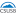California State University, San Bernardino

Partial Representations For Ternary Matroids, Ebony Perez

Electronic Theses, Projects, and Dissertations

In combinatorics, a matroid is a discrete object that generalizes various notions of dependence that arise throughout mathematics. All of the information about some matroids can be encoded (or represented) by a matrix whose entries come from a particular field, while other matroids cannot be represented in this way. However, for any matroid, there exists a matrix, called a partial representation of the matroid, that encodes some of the information about the matroid. In fact, a given matroid usually has many different partial representations, each providing different pieces of information about the matroid. In this thesis, we investigate when a ...